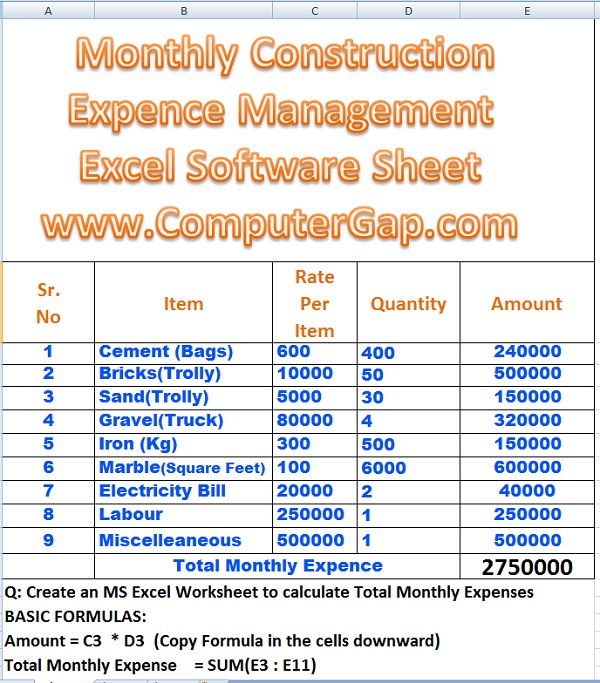# House Building Expenses Formulas in Excel

Today we will discuss the Excel Worksheet Formulas for recording Monthly House Construction Expense Management.

### Steps To Create Expense Management Excel Worksheet

1. First of all, start MS Excel with a new workbook.
2. Merge required number of cells in first row of worksheet.

3. Insert Word Art and type a suitable title heading in worksheet.

5. Enter data in worksheet.
6. Apply the formulas and functions in worksheet as explained later.

Here is the list of expenses we have considered to be recorded.

 Sr. No Item 1 Cement (Bags) 2 Bricks(Trolly) 3 Sand(Trolly) 4 Gravel(Truck) 5 Iron (Kg) 6 Marble(Square Feet) 7 Electricity Bill 8 Labour 9 Miscelleaneous

You can increase this list of expenses according to your wish. The Excel worksheet formulas are provided for the worksheet as shown in the figure below:Monthly House Building Expenses Calculator Formulas in Excel

If you change the worksheet, it is not difficult to change the construction management formulas in Excel according to your expense list.

### The formulas used in Construction Expense Management Excel Worksheet

The formulas used in Construction Expense Management Excel Worksheet are given as follows:
Excel Formula No. 1:
Amount = C3 * D3
Explanation:
First formula will be used to multiply Rate per Item Column Cell with Quantity Column Cell. We can easily check from the above figure of home expenses worksheet that the related column cells are C3 and D3. We use multiplication operator * to multiply these cell values. Don’t forget to place = operator before typing the formula. We will insert formula by typing =C3 * D3 in cell E3. Remember that cell E3 is in the Amount (E) column.

= C3 * D3

#### How To Copy Formula in the Cells Below

• Type the formula in cell E3.
• Point to the lower right corner of this selected cell E3.
• The cursor will take the Plus sign shape.
• Now keeping the left button pressed, drag the mouse downward selecting the cells you wish to copy formula in.
• Release the left button of mouse.
• You will see that formula has been copied and the results of formula appeared in the cells.Calculate Monthly Building Expenses by Formulas in Excel Worksheet

#### Excel Formula for Calculating Total Amount of All Expense

Type   = SUM(E3 : E11) in cell E12 and press Enter key. The formula will produce the resultant value. This result gives us the total monthly expense during a given month.Total Expenses during month by excel formulas
The total monthly expense  is shown below: